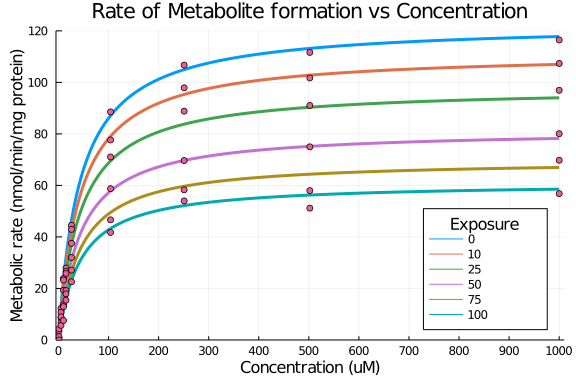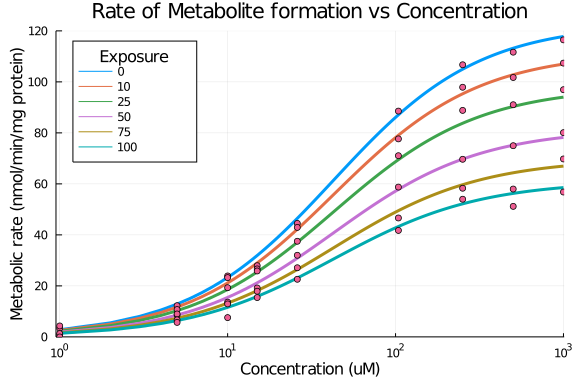# Exercise PK44_2 - Estimation of inhibitory constant Ki

### Background

• Structural model - Estimation of inhibitory rate constant in non-competitive enzyme inhibition model

• Number of subjects - 1

• Number of componds - 1

### Learning Outcome

• To get an understanding about inhibitory models

• To understand the relationship between rate of metabolite formation and concentration

### Objectives

• To analyze enzyme data by means of simulteneous nonlinear regression

### Libraries

call the necessary 'libraries' to get started

using Pumas
using Plots
using CSV
using StatsPlots
using Random


### Model - Non-Competitive Inhibitory Model

pk_44_nim  = @model begin
@param begin
tvvmax ∈ RealDomain(lower=0)
tvkm   ∈ RealDomain(lower=0)
tvki   ∈ RealDomain(lower=0)
Ω      ∈ PDiagDomain(2)
end

@random begin
η      ~ MvNormal(Ω)
end

@covariates conc I

@pre begin
Vmax   = tvvmax * exp(η)
Km     = tvkm * exp(η)
Ki     = tvki
_conc  = conc
_I     = I
end

@derived begin
## Noncompetitive Inhibition Model
rate_nim     = @. ((Vmax * _conc/(Km + _conc)) * (Ki / (Ki + _I)))
end
end

PumasModel
Parameters: tvvmax, tvkm, tvki, Ω, σ_add
Random effects: η
Covariates: conc, I
Dynamical variables:
Derived: rate_nim, dv_rate_nim
Observed: rate_nim, dv_rate_nim


### Parameters

Parameters provided for simulation are as below.tv represents the typical value for parameters.

• Vmax - Maximum metabolic rate (μM*gm_protein/min)

• Km - Michaelis-Mentons constant (μmol/L)

• Ki - Inhibitory constant (μmol/L)

• I - Inhibitor concentration/Exposure

param = ( tvvmax = 122.827,
tvkm   = 42.7053,
tvki   = 98.8058,
Ω      = Diagonal([0.0,0.0]),

(tvvmax = 122.827, tvkm = 42.7053, tvki = 98.8058, Ω = [0.0 0.0; 0.0 0.0],


### Creating a Dataset

In this dataset we will be having time, concentration data and Exposure(I) which will be used to estimate the rate of metabolite concentration

df_sub1 = map(i -> DataFrame(id=i,time = 1:1:1000, dv_rate_nim = missing, conc = 1:1:1000, I=0), 1:6)
df      = vcat(DataFrame.(df_sub1)...)
df[(df[:id] .== 2), :I] = 10
df[(df[:id] .== 3), :I] = 25
df[(df[:id] .== 4), :I] = 50
df[(df[:id] .== 5), :I] = 75
df[(df[:id] .== 6), :I] = 100
df_sub1 = df
sub1    = read_pumas(df_sub1, observations=[:dv_rate_nim], covariates=[:conc, :I], event_data=false)

Population
Subjects: 6
Covariates: conc, I
Observables: dv_rate_nim


### Simulation

We will now simulate the rate of metabolite formation

Random.seed!(123)
sim_sub1 = simobs(pk_44_nim, sub1, param)
df1      = DataFrame(sim_sub1)


### Dataframe and Plot

Use the DataFrame for plotting

##### Non-Competitive Inhibitory Model
df1_dv = filter(x -> x.conc in [1,5,10,15,26,104,251,502,1000], df1)

@df df1 plot(:conc, :rate_nim, group=:I,
title = "Rate of Metabolite formation vs Concentration",
xlabel = "Concentration (uM)", ylabel = "Metabolic rate (nmol/min/mg protein)",
linewidth=3, legend=:bottomright, labeltitle="Exposure",
xticks=[0,100,200,300,400,500,600,700,800,900,1000], xlims=(-5,1010),
yticks=[0,20,40,60,80,100,120], ylims=(0,120))
@df df1_dv scatter!(:conc, :dv_rate_nim, label=false)@df df1 plot(:conc, :rate_nim, group=:I, xaxis=:log,
title = "Rate of Metabolite formation vs Concentration", labeltitle="Exposure",
xlabel = "Concentration (uM)", ylabel = "Metabolic rate (nmol/min/mg protein)",
linewidth=3, legend=:topleft, xticks=[1,10,100,1000], xlims=(0.95,1010),
yticks=[0,20,40,60,80,100,120], ylims=(0,120))
@df df1_dv scatter!(:conc, :dv_rate_nim, label=false)